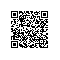# PHP加密扩展库mcrypt安装及应用技巧

PHP程序员们在编写代码程序时，除了要保证代码的高性能之外，还有一点是非常重要的，那就是程序的安全性保障。PHP除了自带的几种加密函数外，还有功能更全面的PHP加密扩展库Mcrypt和Mhash。

1.PHP加密扩展库Mcrypt安装

2.PHP加密扩展库Mcrypt的算法和加密模式

Mcrypt库支持20多种加密算法和8种加密模式，具体可以通过函数mcrypt_list_algorithms()和mcrypt_list_modes()来显示，结果如下：

Mcrypt支持的算法有：cast-128 gost rijndael-128 twofish arcfour cast-256 loki97 rijndael-192 saferplus wake blowfish-compat des rijndael-256 serpent xtea blowfish enigma rc2 tripledes

Mcrypt支持的加密模式有：cbc cfb ctr ecb ncfb nofb ofb stream

DES算法表示为MCRYPT_DES;
ECB模式表示为MCRYPT_MODE_ECB；

3.PHP加密扩展库Mcrypt应用

[php] view plaincopy

1. < ?php  $str = "我的名字是？一般人我不告诉他！"; //加密内容$key = "key:111";   //密钥  $cipher = MCRYPT_DES; //密码类型$modes = MCRYPT_MODE_ECB;  //密码模式  $iv = mcrypt_create_iv(mcrypt_get_iv_size($cipher,$modes),MCRYPT_RAND);//初始化向量 echo "加密明文：".$str."<p>";  $str_encrypt = mcrypt_encrypt($cipher,$key,$str,$modes,$iv);  //加密函数  echo "加密密文：".$str_encrypt." <p>";$str_decrypt = mcrypt_decrypt($cipher,$key,$str_encrypt,$modes,$iv); //解密函数 echo "还原：".$str_decrypt;  ?>

<1>由例子中可看到，使用PHP加密扩展库Mcrypt对数据加密和解密之前，首先创建了一个初始化向量，简称为iv。由 $iv = mcrypt_create_iv(mcrypt_get_iv_size($cipher,$modes),MCRYPT_RAND);可见创建初始化向 量需要两个参数：size指定了iv的大小；source为iv的源，其中值MCRYPT_RAND为系统随机数。 <2>函数mcrypt_get_iv_size($cipher,$modes)返回初始化向量大小，参数cipher和mode分别指算法和加 密模式。 <3>加密函数$str_encrypt = mcrypt_encrypt($cipher,$key,$str,$modes,$iv); 该函数的5个参数分 别如下：cipher——加密算法、key——密钥、data(str)——需要加密的数据、mode——算法模式、 iv——初始化向量 <4>解密函数 mcrypt_decrypt($cipher,$key,$str_encrypt,$modes,$iv); 该函数和加密函数的参数几乎 一样，唯一不同的是data，也就是说data为需要解密的数据$str_encrypt，而不是原始数据$str。使用钉钉扫一扫加入圈子
+ 订阅